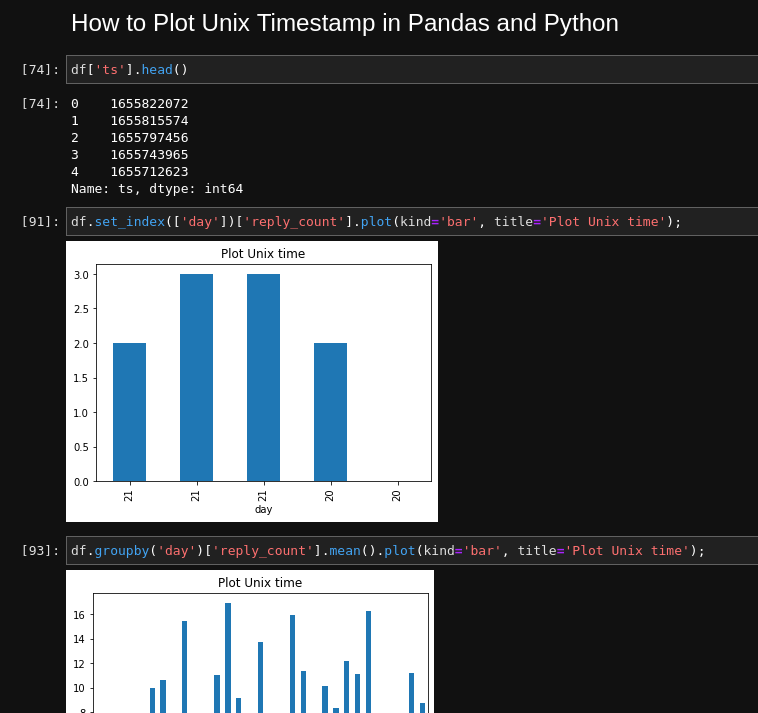In this short article we will see how to plot Unix timestamp in Pandas and Python.

To plot readable dates we will do a basic conversion of Unix timestamp to date in Pandas. To find more detailed examples visit How to Convert Unix Time to Date in Pandas

## Setup

Let's create a DataFrame with Unix timestamp information:

``````dict = {'ts': {0: 1655822072.437469,
1: 1655815574.333629,
2: 1655797456.516109,
3: 1655743965.358579,
4: 1655712623.707739},
'reply_count': {0: 2.0, 1: 3.0, 2: 3.0, 3: 2.0, 4: None}}

pd.DataFrame(dict)
``````

Final data:

0 1655822072.437469 2.0
1 1655815574.333629 3.0
2 1655797456.516109 3.0
3 1655743965.358579 2.0
4 1655712623.707739 NaN

## Step 1: Convert Unix timestamp to date

First we will convert the Unix time to Pandas datetime by:

``````df['date'] = pd.to_datetime(df['date'],unit='s')
``````

The result will be timestamp:

``````1655822072.437469
``````

to datetime:

``````DatetimeIndex(['2022-06-21 14:34:32.437469006',
'2022-06-21 12:46:14.333628893',
'2022-06-21 07:44:16.516108990'],
dtype='datetime64[ns]', freq=None)
``````

## Step 2: Convert datetime to readable date

Next we will extract readable dates from the datetime in order to plot them.

We can get any date format(if needed consult with: How to Convert Unix Time to Date in Pandas.

For simplicity we will use day:

``````df['day'] = df['date'].dt.day
``````

This will create new column which has day of month for a given time stamp

## Step 3: Plot Unix timestamp as index

We can plot a given value for each day. In DataFrame above we have pairs of `date` and `quantity`:

``````2 - 2022-06-21
3 - 2022-06-21
``````

To plot this information we can set the `day` or `date` column as index. Then select the column which needs to be plotted by:

``````df.set_index(['day'])['reply_count'].plot(title='Plot Unix time');
``````

We can select also the type of the plot by `kind='bar'`.

## Step 4: Group by Unix timestamp date and plot

Finally suppose we would like to get statistic per each period: day, week, month etc.

For this purpose we can convert the datetime to each time unit. We need also to group by each unit.

Finally we need to select a column and function which should be applied like:

• `count()`
• `sum()`
• `mean()`
``````df.groupby('day')['reply_count'].count().plot(kind='bar', title='Plot Unix time');
``````